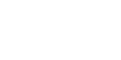The calculation of loops and rows at the beginning of the binding. A significant difficulty encountered when establishing the number of loops as at the beginning of work and further define the width of the garment and sleeves. Commonly the number of loops determined by eye, but it is quite wrong and inaccurate.

After you establish the width and length of such a sample is not difficult to establish the number of stitches and rows needed to obtain the desired sizes. Experience shows that the definition of size in the loops and rows of this model is not always accurate. In both cases, the discrepancy is a result of the dissimilarity of knitting - sometimes it is more free and sometimes more dense, different thickness of yarn, etc. So that the product and precise measurements, you must first prepare a pattern and use it. To calculate the exact number of loops, it is necessary to link the square of about 20 loops and 20 rows, well it is ironed, then a very simple way to determine the number of loops required for the initial series of this knitwear. The calculation of loops is as follows. Ordinary cardboard should be cut square and in the middle of it is another smaller square (5 X 5 cm) on the sides of the square centimeter is applied to division. If there is no such square, you can use an ordinary centimeter, which is applied to the knitted square first in the transverse direction. With pins mark the distance and set the number of stitches per one centimeter. After that, the cm is applied in the longitudinal direction, and again using the pins fix the number of ranks per one centimeter.

After you establish the width and length of such a sample is not difficult to calculate the number of stitches and rows needed to obtain the desired sizes.